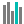#BINOM.INV (PQL - xl)

This function returns the criterion binomial, the smallest value for which the cumulative binomial distribution is greater than or equal to a criterion value

• Library: PQL \ Spreadsheet \ Statistical
• Compatibility: Any content (regardless of data source) in the Tabulate spreadsheet module
• Solve: This function can be used with the Solve plug-in: both as part of the objective function and constraint functions

#### Syntax

BINOM.INV(trials, probability s, alpha)

##### Function Arguments
 Name Description Type Optional trials Number of trials; if not an integer, the number is truncated Number probability s Probability of success on each trial; number between 0 and 1 Number alpha Alpha, value for the criterion Number